# How Many Gallons Of Water Are In My Pool

Written on:April 30, 2022

### How Many Gallons Of Water Are In My Pool ?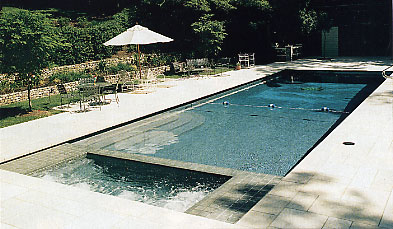In order to figure out the proper doses of chemicals for your pool, you need to determine how many gallons of water it holds. To do that, you need to use a ruler to measure your pool to find out these four different numbers; Length, Width, Average depth and a multiplier that determines gallons.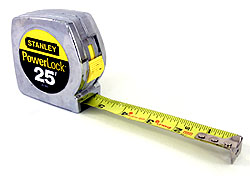So here are the formulas to figure out how much water is in your swimming pool:
These formulas hold true for both Inground pools as well as above ground pools.

Rectangular, Square or Free Form Pools:

Length x Width x Average Depth x 7.5 = Total gallons

For example: 16′ x 32′ x 4.5′ x 7.5 = 17,280 Gallons

Circular Pools:

Diameter x Diameter x Average Depth x 5.9 = Total gallons

For example: 18′ x 18′ x 4′ x 5.9 = 7,646 Gallons

Oval Pools:

Length x Width x Average Depth x 5.9 = Total gallons

For example: 15′ x 30′ x 4′ x 5.9 = 10,620 Gallons

How to determine the Average Depth of a Swimming Pool where the bottom slopes:

Below is a side-shot of the “typical” inground pool that has a shallow end (see “D”), then a slope that leads into the deep end (see “C”).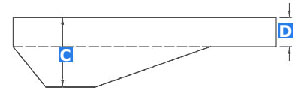First measure the depth of “D”, the shallow end (usually anywhere from 3′ to 5′), and then measure the depth of “C”, the deep end (usually anywhere between 6′ and 12′) . Then, add them together and divide by 2. This is the average depth of your pool.

For example, if your shallow end “D” is 3′, and your deep end “C” is 8′, this would be your formula for the Average Depth:

3′ + 8′ = 11′

11′ divided by 2 = 5.5.

Therefore, in this example, your average depth would be 5 1/2 feet.

Most Common Sizes Of Inground Rectangular Pools

Below, we have created some handy charts of the most popular sizes of pools
and how many gallons of water are in them.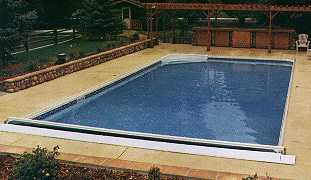POOL SIZE 4′ AVG. DEPTH 4.5′ AVG. DEPTH 5′ AVG. DEPTH 5.5′ AVG. DEPTH 6′ AVG. DEPTH 12′ X 24′ 8,640 9,720 10,800 11,880 12,960 14′ X 28′ 11,760 13,230 14,700 16,170 17,640 15′ X 30′ 13,500 15,200 19,900 18,600 20,250 16′ X 32′ 15,400 17,300 19,200 21,100 23,040 18′ X 36′ 19,400 21,900 24,300 26,700 29,160 20′ X 40′ 24,000 27,000 30,000 33,000 36,000 25′ X 45′ 33,750 37,968 42,187 46,406 50,625 25′ X 50′ 37,500 42,200 46,900 51,600 56,250 30′ X 50′ 45,000 50,525 56,250 61,875 67,500

Most Common Sizes Of Above Ground Pools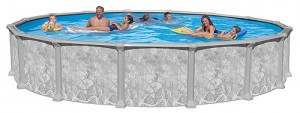### Above Ground Pools with a Depth of 48″ (4′)

 SIZE GALLONS OF WATER 12′ Round 3,398 15′ Round 5,310 18′ Round 7,646 21′ Round 10,407 24′ Round 13,593 27′ Round 17,204 28′ Round 18,502 30′ Round 21,240 33′ Round 25,700 15′ x 30′ Oval 10,620

### Above Ground Pools with a Depth of 52″ (4.5′)

 SIZE GALLONS OF WATER 12′ Round 3,823 15′ Round 5,973 18′ Round 8,602 21′ Round 11,708 24′ Round 15,292 27′ Round 19,354 28′ Round 20,815 30′ Round 23,895 33′ Round 28,912 15′ x 30′ Oval 11,947